### Test (Brandon)

Scalars and vectors are mathematical objects that are used to quantify physical quantities.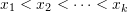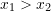Nulla vitae elit libero, a pharetra augue. Cras mattis consectetur purus sit amet fermentum. Maecenas sed diam eget risus varius blandit sit amet non magna.

Scalar. A scalar is a real number. For example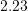,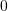,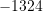,, and so on .

### EXAMPLE asdfasfd

Vestibulum id ligula porta felis euismod semper. Maecenas sed diam eget risus varius blandit sit amet non magna.Vestibulum id ligula porta felis euismod semper. Maecenas sed diam eget risus varius blandit sit amet non magna.

Vestibulum id ligula porta felis euismod semper. Maecenas sed diam eget risus varius blandit sit amet non magna.

Scalar quantity. A scalar quantity is a quantity that can be described by a single real number. This number specifies the magnitude or size of that quantity. For example length, angle, mass, speed, area, temperature, and pressure are scalar quantities.

Nulla vitae elit libero, a pharetra augue. Cras mattis consectetur purus sit amet fermentum. Maecenas sed diam eget risus varius blandit sit amet non magna.

Sometimes a scalar quantity is simply referred to as scalar. For example, “mass is a scalar” is equivalent to stating: “mass is a scalar quantity”.

The mathematical operations on scalars follow the usual rules of arithmetic.

Vestibulum id ligula porta felis euismod semper. Maecenas sed diam eget risus varius blandit sit amet non magna.

Vector. A vector is a mathematical object that has a size (magnitude) and a direction. This definition perfectly fulfills the requirements of engineering mechanics. However, for a more rigorous mathematical definition, please refer to section 2.8.

Vector quantity. A vector quantity is a quantity characterized by both a magnitude and a direction. For example velocity, force, acceleration, and moment are all vector quantities.

Vestibulum id ligula porta felis euismod semper. Maecenas sed diam eget risus varius blandit sit amet non magna.

Curabitur blandit tempus porttitor. Integer posuere erat a ante venenatis dapibus posuere velit aliquet. Nullam id dolor id nibh ultricies vehicula ut id elit.

The magnitude (sometimes referred to as the norm) of a vector is a positive scalar. For example the magnitude of the velocity of a moving object is the speed of that object. Speed is always measured and reported as a positive number. The magnitude of a physical quantity must have a unit.

The line that is collinear with an arrow is called the line of action. The orientation or direction of an arrow (its tail is already known or defined) with respect to a fixed axis is defined by an angle formed between the axis and the line of action of the arrow. Fig. 2.2 shows a directed line segment or arrow representing the vector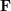with its defined terms.

Zero vector. The zero vector, denoted by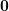or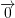, is a vector of length zero. The zero vector does not point toward any direction, therefore, its direction is undefined.